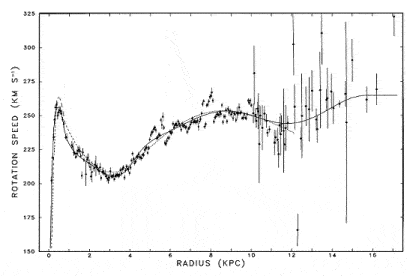# Different speed of light at different milky way radius?

• Juanma

#### Juanma

The rotation speed of planets around the sun follows a simple rule: if you double the distance to the sun the rotation speed is reduced by the square root of 2. No matter the mass of the planet. If Earth mass was different the period of rotation around the sun will be exactly the same.

But in the milky way the rotation speed of stars around it's center does not follow this rule.
This is a map of velocities of stars around the milky way:May this variance of speed be an effect and not the real motion?

You may have heard that light slows down when passing throuhg superfluids. By use of a Bose-Einstein condensate, Danish physicist Lene Vestergaard Hau (Harvard University) succeeded in slowing a beam of light to about 17 metres per second, and, in 2001, was able to momentarily stop a beam. ()

So what if the temperature of the interestelar medium is different at different radius? So the speed of light at our radius could be 299792458 m/s but the light coming from different radius may travel through a medium at lower temperature and slower speed. This could explain why we see that the radial motion of the milky does not follow Kepler's third law.

Last edited by a moderator:

In the solar system, more than 99% of the total mass is located in the center (the sun), this gives the simple relation between distance and orbital velocity.
In the milky way, most of the mass is located in the disk, spread out over the whole galaxy. This leads to a more complicated distance<->orbital velocity relation.

May this variance of speed be an effect and not the real motion?
An effect of what?
The speed of light has nothing to do with that. While its speed does depend on the medium, the variations are extremely small in the interstellar medium, and it does not influence gravity at all.

This could explain why we see that the radial motion of the milky does not follow Kepler's third law.

Only as a pie in the sky speculation...try some calculations and you'll find the hypothesis
would not work under some reasonable temperature variation assumptions. Nevertheless,
such dream scenarios can be fun to contemplate.

You might find the work astronomer Vera Rubin of interest.